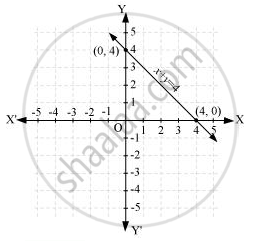Share

# Draw the graph of the following linear equations in two variables:- x + y = 4 - CBSE Class 9 - Mathematics

ConceptGraph of a Linear Equation in Two Variables

#### Question

Draw the graph of the following linear equations in two variables:- x + y = 4

#### Solution

It can be observed that x = 0, y = 4 and x = 4, y = 0 are solutions of the above equation. Therefore, the solution table is as follows.

 x 0 4 y 4 0

The graph of this equation is constructed as follows.Is there an error in this question or solution?

#### APPEARS IN

NCERT Solution for Mathematics Class 9 (2018 to Current)
Chapter 4: Linear Equations in two Variables
Ex. 4.30 | Q: 1.1 | Page no. 74

#### Video TutorialsVIEW ALL 

Solution Draw the graph of the following linear equations in two variables:- x + y = 4 Concept: Graph of a Linear Equation in Two Variables.
S